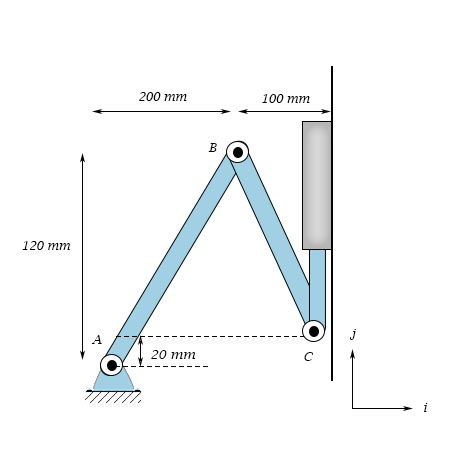# Consider the linkage system below. At the instance shown, point C moves downward at 1.5 m/s and...

## Question:

Consider the linkage system below. At the instance shown, point {eq}C {/eq} moves downward at {eq}1.5 m/s {/eq} and has a downward acceleration of {eq}4 m/s^2 {/eq}. Pay attention to the reference axis shown below, and be sure to denote the proper vector notation on all answers.

a) Write down the position vector of point {eq}B {/eq} with respect to point {eq}A {/eq},

b) Write down the position vector of point {eq}C {/eq} with respect to point {eq}B {/eq},

c) Write down the position vector of point {eq}C {/eq} with respect to point {eq}A {/eq},

Using the equation, determine {eq}\omega _{AB} {/eq} and {eq}\omega_{BC} {/eq}, the rotation velocities of the two links. Be sure to properly denote the vector direction of the rotational velocity.

Write down a vector equation relationship between the linear acceleration at point {eq}C {/eq} and the linear acceleration at point {eq}A {/eq}. Using this equation, determine {eq}\alpha_{AB} {/eq} and {eq}\alpha_{BC} {/eq}, the rotational acceleration of the two links. Be sure to properly denote the vector directions.## Vector:

Vector are characterized by its magnitude and direction such as velocity, acceleration,force and momentum.Vector quantity is only depends on magnitude and direction of the particle. In this question we have to find the direction and magnitude of particles about x-axis.

Given Data:

• In the given figure the linkage shown at point C moves in downward direction with speed 1.5 m/s.
• And it has downward acceleration of {eq}4\;m/{s^2} {/eq}
• From given figure we have the coordinates as {eq}A\left( {0,0} \right),B\left( {200,120} \right),C\left( {300,20} \right) . {/eq}

1.1) The position vector of B with respect to A is ,

{eq}{r_{B/A}} = {r_B} - {r_A} {/eq}

Substitute the value of coordinates we get ,

{eq}\begin{align*} {r_{B/A}} &= \left( {200 - 0} \right)i + \left( {120 - 0} \right)j\\ &= 200i + 120j \end{align*} {/eq}

1.2) Position vector of C with respect to B.

{eq}\begin{align*} {r_{C/B}} &= {r_C} - {r_B}\\ {r_{B/A}} &= \left( {300 - 200} \right)i + \left( {20 - 120} \right)j\\ &= 100i + 100j \end{align*} {/eq}

1.3) Position vector of C with respect to A.

{eq}\begin{align*} {r_{C/A}} &= {r_C} - {r_A}\\ {r_{B/A}} &= \left( {300 - 0} \right)i + \left( {20 - 0} \right)j\\ {r_{B/A}} &= 300i + 20j \end{align*} {/eq}

• Now the vector relationship equation is :

{eq}{V_{C/A}} = {V_{C/B}} - {V_{B/A}} {/eq}

here we have given that,

{eq}\begin{align*} {V_{C/A}} &= - 1.5j\;{\rm{m/s}}\\ {V_{C/A}} &= - 1500j\;{\rm{mm/s}} \end{align*} {/eq}

Also,

{eq}\begin{align*} {V_{C/A}} &= {r_{C/B}} \times {\omega _{BC}}\\ {V_{B/A}} &= {r_{B/A}} \times {\omega _{AB}} \end{align*} {/eq}

Substitute the values in above equation we get ,

{eq}\begin{align*} - 1500j &= \left( {100i - 100j} \right) \times \left( {{\omega _{BC}}} \right) + \left( {200i + 120j} \right)\left( {{\omega _{AB}}} \right)\\ - 1500j &= \left( { - 100 \times {\omega _{BC}}i - 100 \times {\omega _{BC}}j} \right) + \left( {120 \times {\omega _{AB}}i - 200 \times {\omega _{AB}}j} \right)\\ - 1500j &= \left( {120 \times {\omega _{AB}} - 100 \times {\omega _{BC}}} \right)i - \left( {200 \times {\omega _{AB}} - 100 \times {\omega _{BC}}} \right)j \end{align*} {/eq}

Comparing i and j components on both sides ,

then i components are ,

{eq}120 \times {\omega _{AB}} - 100 \times {\omega _{AB}} = 0 {/eq}

j components are,

{eq}- \left( {200 \times {\omega _{AB}} - 100 \times {\omega _{BC}}} \right) = - 1500 {/eq}

On solving both equation we get

{eq}\begin{align*} 320 \times {\omega _{AB}} &= - 1500\\ {\omega _{AB}} &= 4.687\;{\rm{rad/s}} \end{align*} {/eq}

and {eq}{\omega _{BC}} = 5.625\;{\rm{rad/s}} {/eq}